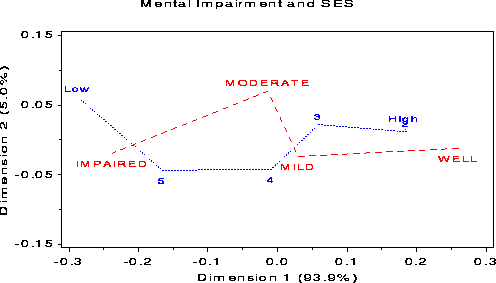corresp Correspondence analysis of contingency tables corresp

# Visualizing Categorical Data: corresp

\$Version: 1.9-1 (27 Feb 2013)
Michael Friendly
York University

## The corresp macro (get corresp.sas)

### Correspondence analysis of contingency tables

The CORRESP macro carries out simple correspondence analysis of a two-way contingency table, and various extensions (stacked analysis, MCA) for a multiway table, as in the CORRESP procedure. It also produces labeled plots of the category points in either 2 or 3 dimensions, with a variety of graphic options, and the facility to equate the axes automatically.

The macro takes input in one of two forms:

(a)
A two-way contingency table, where the columns are separate variables and the rows are separate observations (identified by a row ID variable. For this form, specify:
```     ID=ROWVAR, VAR=C1 C2 C3 C4 C5
```
(b)
A contingency table in frequency form (e.g., the output from PROC FREQ), or raw data, where there is one variable for each factor. In frequency form, there will be one observation for each cell. For this form, specify:
```     TABLES=A B C
```

Include the WEIGHT= parameter when the observations are in frequency form.

## Usage

The CORRESP macro is called with keyword parameters. Either the VAR= parameter or the TABLES= parameter (but not both) must be specified, but other parameters or options may be needed to carry out the analysis you want. The arguments may be listed within parentheses in any order, separated by commas. For example:
```  %corresp(var=response, id=sex year);
```
The plot may be re-drawn or customized using the output OUT= data set of coordinates and the ANNO= Annotate data set.

### Parameters

DATA=
Specifies the name of the input data set to be analyzed. [Default: `DATA=_LAST_`]
VAR=
Specifies the names of the column variables for simple CA, when the data are in contingency table form. Not used for MCA.
ID=
Specifies the `name(s)` of the row `variable(s)` for simple CA. Not used for MCA.
TABLES=
Specifies the names of the factor variables used to create the rows and columns of the contingency table. For a simple CA or stacked analysis, use a ',' or '/' to separate the the row and column variables.
WEIGHT=
Specifies the name of the frequency (WEIGHT) variable when the data set is in frequency form. If WEIGHT= is omitted, the observations in the input data set are not weighted.
SUP=
Specifies the `name(s)` of any variables treated as supplementary. The categories of these variables are included in the output, but not otherwise used in the computations. These must be included among the variables in the VAR= or TABLES= option.
DIM=
Specifies the number of dimensions of the CA/MCA solution. Only two dimensions are plotted by the PPLOT option, however.
OPTIONS=
Specifies options for PROC CORRESP. Include `MCA` for an MCA analysis, `CROSS=ROW|COL|BOTH` for stacked analysis of multiway tables, `PROFILE=BOTH|ROW|COLUMN` for various coordinate scalings, etc. [Default: `OPTIONS=SHORT`]
OUT=
Specifies the name of the output data set of coordinates. [Default: `OUT=COORD`]
ANNO= Specifies the name of the annotate data set of labels produced by the macro. [Default: `ANNO=LABEL`]
PPLOT=
Produce printer plot? [Default: `PPLOT=NO`]
GPLOT=
Produce graphics plot? [Default: `GPLOT=YES`]
PLOTREQ=
The dimensions to be plotted [Default: `PLOTREQ=DIM2*DIM1` when `DIM=2`, `PLOTREQ=DIM2*DIM1=DIM3` when `DIM=3`]
HTEXT=
Height for row/col labels. If not specified, the global HTEXT goption is used. Otherwise, specify one or two numbers to be used as the height for row and column labels. The HTEXT= option overrides the separate ROWHT= and COLHT= parameters (maintained for backward compatibility).
ROWHT=
Height for row labels
COLHT=
Height for col labels
COLORS=
Colors for row and column points, labels, and interpolations. [Default: `COLORS=BLUE RED`]
POS=
Positions for row/col labels relative to the points. [Default: `POS=5 5`]
SYMBOLS=
Symbols for row and column points [Default: `SYMBOLS=NONE NONE`]
INTERP=
Interpolation options for row/column points. In addition to the standard interpolation options provided by the SYMBOL statement, the CORRESP macro also understands the option VEC to mean a vector from the origin to the row or column point. [Default: `INTERP=NONE NONE`, `INTERP=VEC` for MCA]
HAXIS=
AXIS statement for horizontal axis. If both HAXIS= and VAXIS= are omitted, the program calls the EQUATE macro to define suitable axis statements. This creates the axis statements AXIS98 and AXIS99, whether or not a graph is produced.
VAXIS=
AXIS statement for vertical axis- use to equate axes
VTOH=
The vertical to horizontal aspect ratio (height of one character divided by the width of one character) of the printer device, used to equate axes for a printer plot, when `PPLOT=YES`. [Default: `VTOH=2`]
INC=
X, Y axis tick increments (for the EQUATE macro). Ignored if HAXIS= and VAXIS= are specified. [Default: `INC=0.1 0.1`]
XEXTRA=
The number of extra X axis tick marks at left and right. Use to allow extra space for labels. [Default: `XEXTRA=0 0`]
YEXTRA=
The number of extra Y axis tick marks [Default: `YEXTRA=0 0`]
M0=
Length of origin marker, in data units. [Default: `M0=0.05`]
DIMLAB=
Prefix for dimension labels [Default: `DIMLAB=Dimension` when `DIM=2`, otherwise, `DIMLAB=Dim`]
NAME=
Name of the graphics catalog entry [Default: `NAME=corresp`]

### Example

```%include vcd(corresp);        *-- or include in an autocall library;
%include data(mental);
axis1 length=3 in  order=(-.15 to .15 by .10)
label=(h=1.5 a=90 r=0);
axis2 length=6 in  order=(-.30 to .30 by .10)
label=(h=1.5) offset=(1);
%corresp (data=mental, id=ses, var=Well Mild Moderate Impaired,
vaxis=axis1, haxis=axis2, htext=1.3, pos=-, interp=join,
symbols=triangle square);
```## Dependencies

The CORRESP macro calls several other macros not included here. It is assumed these are stored in an autocall library. If not, you'll have to `%include` them in your SAS session or batch program.
• LABEL macro - label points
• EQUATE macro - equate axes